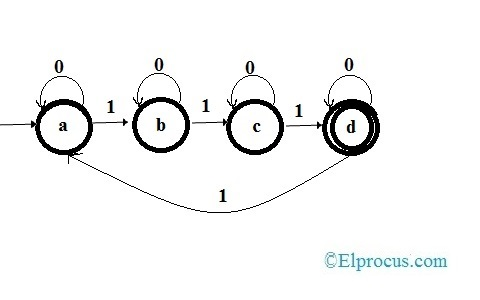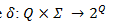# Automata Theory : Terminologies, and Applications

In today’s technological era both hardware and software field has seen tremendous development. One of the major areas of development was seen in the methods of Hardware designs. With the growing technology, the concept of Hardware – Software co-design was possible to implement. Different methods are developed by which, using software one can fully design and simulate the hardware systems. One of such methods is the Automata Theory. Automata theory is the branch of computer science that deals with designing the abstract model of computing devices which follow the predetermined sequence of steps automatically. This article discusses brief information on automata tutorial.

## What is Automata Theory?

The word Automata is derived from Greek, which means “self-acting”. An Automaton is a mathematical model of the machine. Automaton consists of states and transitions. As the input is given to automaton it makes a transition to the next state, depending upon the transition function. The inputs to the transition function are present state and recent symbols. If an Automaton has a finite number of states, it is known as Finite Automata or Finite State Machine. The finite automata are represented by a 5-tuple(Q,∑,δ, qo , F)

Where,

Q= Finite set of states.

∑= finite set of symbols also called Alphabet of the automata.

δ  = the transition function.

qo = initial state of the input.

F= set of final states of Q.

### Basic Terminologies of Automata Theory

Some of the basic terminologies of Automata Theory are-

1. Alphabet: Any finite set of symbols in automata theory is known as Alphabet. Represented by the letter∑ the set {a, b, c, d, e,} is called Alphabet set, whereas the letters of set ‘a’, ‘b’, ‘c’, ‘d’, ‘e’ are called symbols.

2. String: In automata, a string is a finite sequence of symbols taken from the alphabet set ∑, For example, the string S = ‘adeaddadc’ is valid upon the alphabet set∑ = {a, b, c, d,e,}.

3. Length of String: The number of symbols present in the string is known as Length of string. For the string S = ‘adaada’ length of the string is|S| = 6. If the length of the string is 0, then it is called an empty string.

4. Kleen Star: It is the unary operator on the set of symbols Σ, which gives the infinite set of all the possible strings, including λ, of all the possible lengths over the set Σ. It represented by. For example, for the set Σ ={ c, d} ,∑* = { λ , c, d, cd, dc, cc, dd,……}.

5. Kleen Closure: It the infinite set of all the possible strings of the alphabet set excluding λ. It is denoted by. For the set Σ ={ a, d},∑+= { a, d, ad, da, aa, dd,…..}.

6. Language: Language is the subset of the Kleene star set∑* for some Alphabet set Σ. Language can be finite or infinite. For example if a language takes all the possible strings of length 2 over the set Σ = {a, d}, then L = {aa, ad, da, dd}.

### Formal Languages and Automata

In automata theory, Formal language is a set of strings, where each string is composed of symbols belonging to the finite Alphabet set Σ. Let us consider a cat language, which can contain any strings from the below infinite set…
mew!
meww!
mewww!!……

The alphabet set for cat language is Σ = {m, e, w, !}. Let this set be used for a Finite State Automata Model-m. Then the formal language characterized by the model m is denoted by

L(m)
L(m) = {‘mew!’, ‘meww!’, ‘mewww’,……}

Automaton is useful for defining a language because it can express an infinite set in a closed form. Formal languages are not the same as the natural languages we speak in our day to day life. A formal language can denote the different states of the machine, unlike our regular languages. Formal language is used to model a part of the natural language such as syntax etc…Formal languages are defined by finite state automata. There are two main perspectives of Finite state automata- Acceptors that can tell if a string is in the language and the second one is the generator that produces only the strings in the language.

### Deterministic Finite Automata

In Deterministic type of automata, when an input is given, we can always determine to which state the transition would be. As this is a finite automaton, it is called Deterministic Finite Automata.

### Graphical Representation

State Diagram is the digraphs used for graphical representation of Deterministic Finite Automata. Let us understand with an example. Let deterministic finite automata be…
Q ={a, b, c, d}.
Σ = {0, 1}
= {a}
F = {d} and the transition function be

#### State DiagramState Diagram of Deterministic Finite State Automata

From the state diagram

•  The states are represented by vertices.
•  Transitions are represented by the arc labeled with an input alphabet.
•  The empty single incoming arc represents the initial state.
•  The state with double circles is the final state.

### Non Deterministic Finite Automata

The automata where the output state for the given input cannot be determined is called Non-Deterministic Automata. It is also called Non-Deterministic Finite Automata, as it has a finite number of states. Non-deterministic Finite Automata is represented as the set of 5 –tuple where(Q ,∑,δ,qo , F)

Q = finite set of states.
∑= Alphabet set.
δ= the transition function

where:qo = Initial state.

### Graphical Representation

Non-deterministic finite automata are represented with the help of the state diagram. Let the Non-Deterministic Finite Automata be-

Q = {a,b,c,d}
Σ = {0,1}
qo = {a}
F = {d}

The transitions are